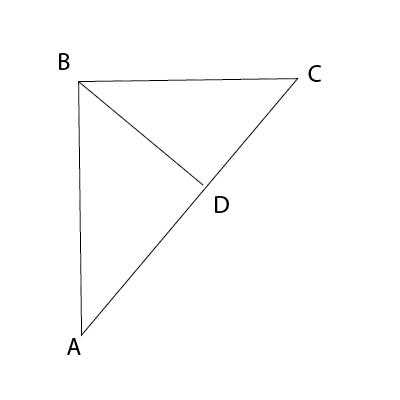### CAT 1999 Question Paper Question 48

Instructions

DIRECTIONS for the following three questions

These questions are based on the situation given below:

A road network (shown in the figure below) connects cities A, B, C and D. All road segments are straight lines. D is the midpoint on the road connecting A and C. Roads AB and BC are at right angles to each other with BC shorter than AB. The segment AB is 100 km long. Ms. X and Mr. Y leave A at 8:00 am, take different routes to city C and reach at the same time. X takes the highway from A to B to C and travels at an average speed of 61.875 km per hour. Y takes the direct route AC and travels at 45 km per hour on segment AD. Y's speed on segment DC is 55 km per hour.Question 48

# What is the average speed of Y in km per hour?

Solution

Y takes the direct route AC and travels at 45 km per hour on segment AD. Y's speed on segment DC is 55 km per hour.

AD=DC =a(D is the midpoint on the road connecting A and C.)

The time taken by Y to reach D= a/45

Further, the time taken by Y to reach C= a/55

Y has travelled equal distances are constant speed.

=> Average speed = $$\frac{2a}{\frac{a}{45}+\frac{a}{55}}$$=$$\frac{2*45*55}{100}$$ = 49.5 kmph